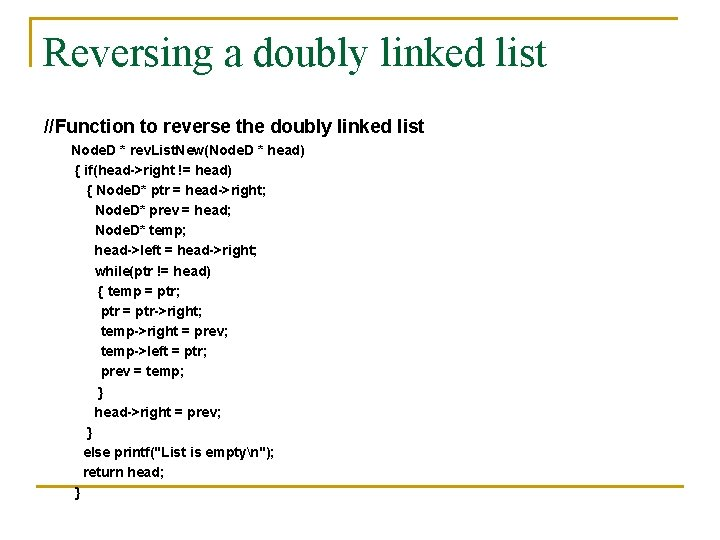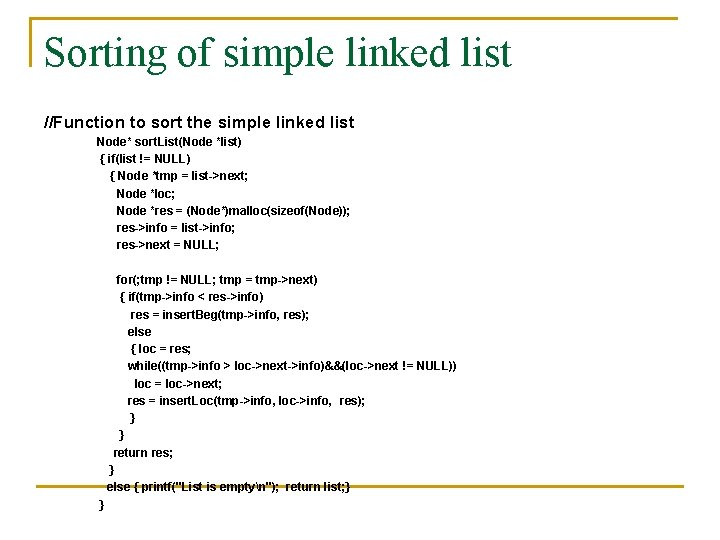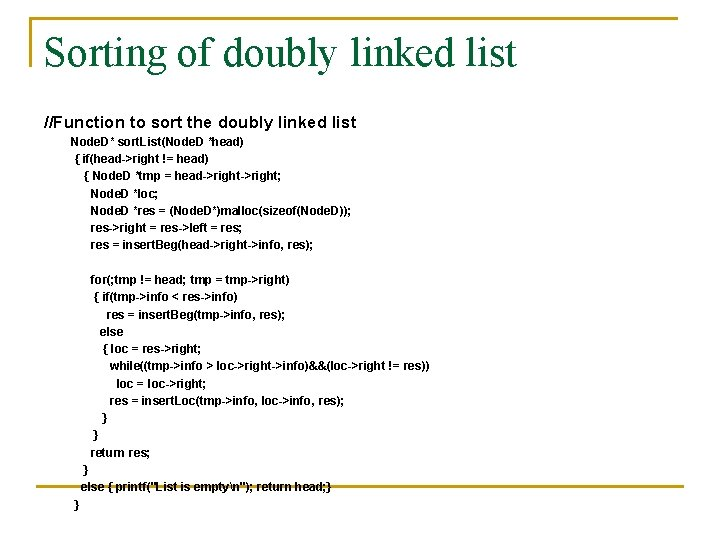• Slides: 41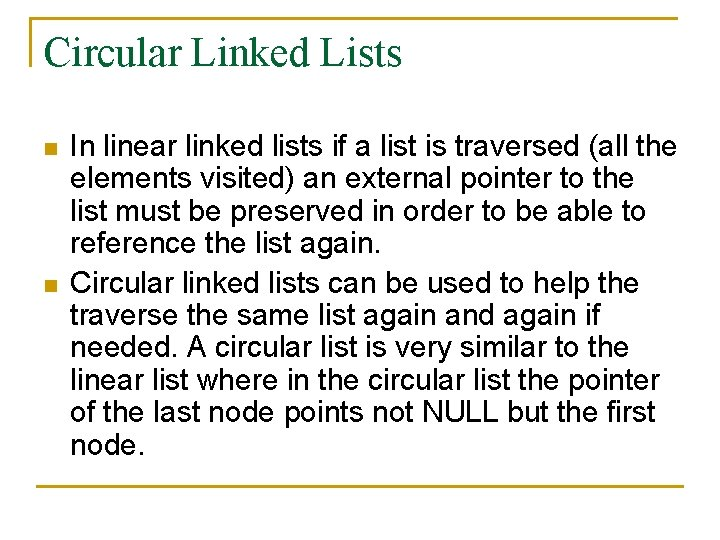Circular Linked Lists n n In linear linked lists if a list is traversed (all the elements visited) an external pointer to the list must be preserved in order to be able to reference the list again. Circular linked lists can be used to help the traverse the same list again and again if needed. A circular list is very similar to the linear list where in the circular list the pointer of the last node points not NULL but the first node.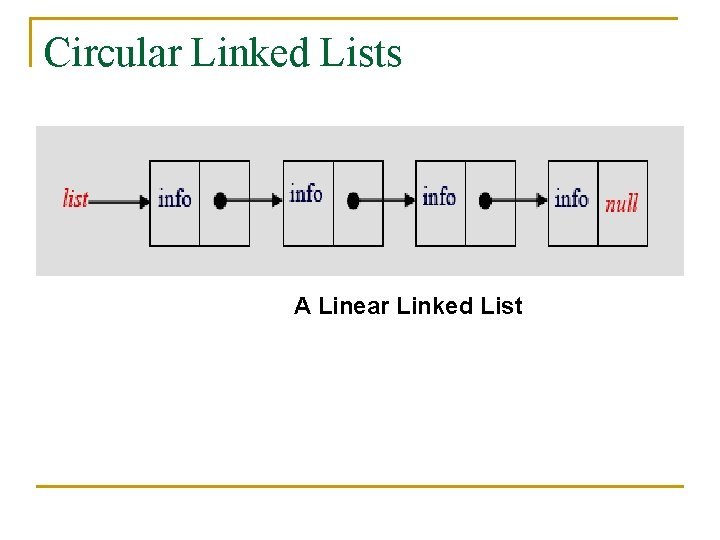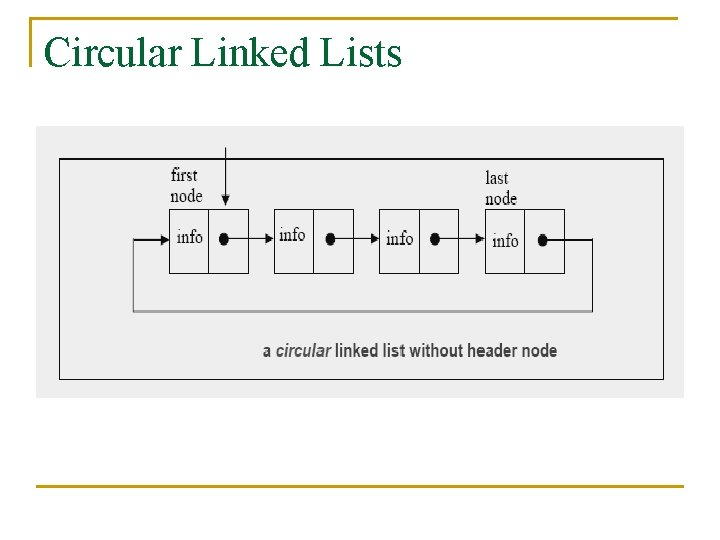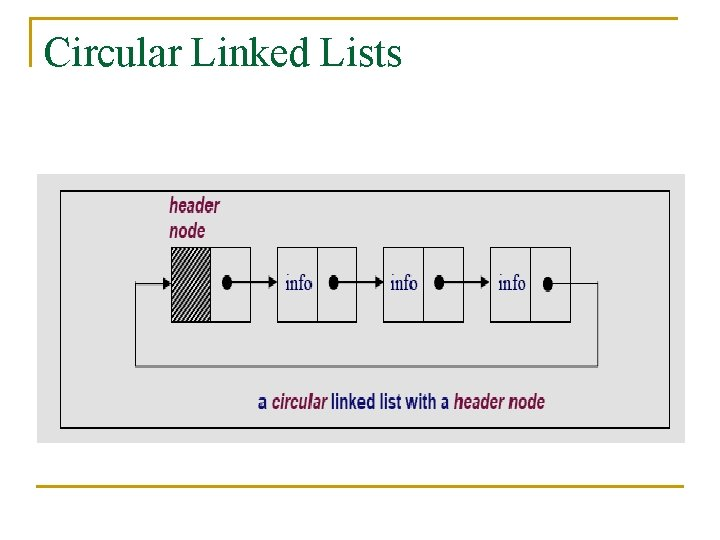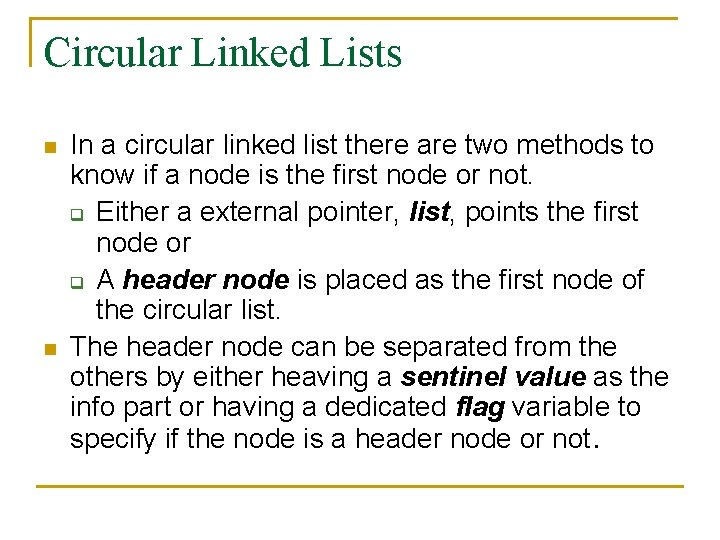Circular Linked Lists n n In a circular linked list there are two methods to know if a node is the first node or not. q Either a external pointer, list, points the first node or q A header node is placed as the first node of the circular list. The header node can be separated from the others by either heaving a sentinel value as the info part or having a dedicated flag variable to specify if the node is a header node or not.CIRCULAR LIST with header node n. The header node in a circular list can be specified by a sentinel value or a dedicated flag: n. Header Node with Sentinel: Assume that info part contains positive integers. Therefore the info part of a header node can be -1. The following circular list is an example for a sentinel used to represent the header node: struct node{ int info; struct node *next; }; typedef struct node *NODEPTR;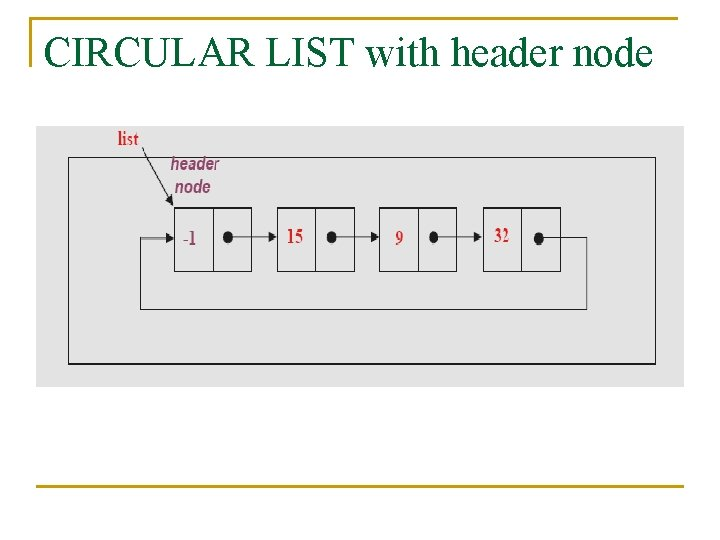CIRCULAR LIST with header node n. Header Node with Flag: In this case a extra variable called flag can be used to represent the header node. For example flag in the header node can be 1, where the flag is 0 for the other nodes. struct node{ int flag; int info; struct node *next; }; typedef struct node *NODEPTR;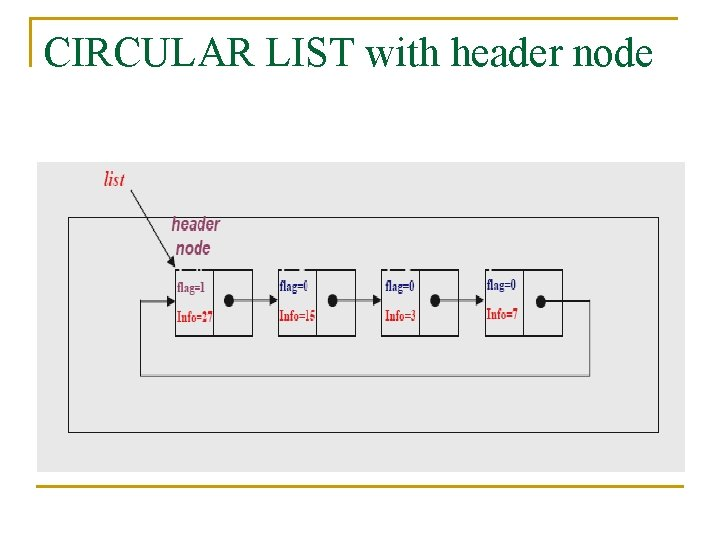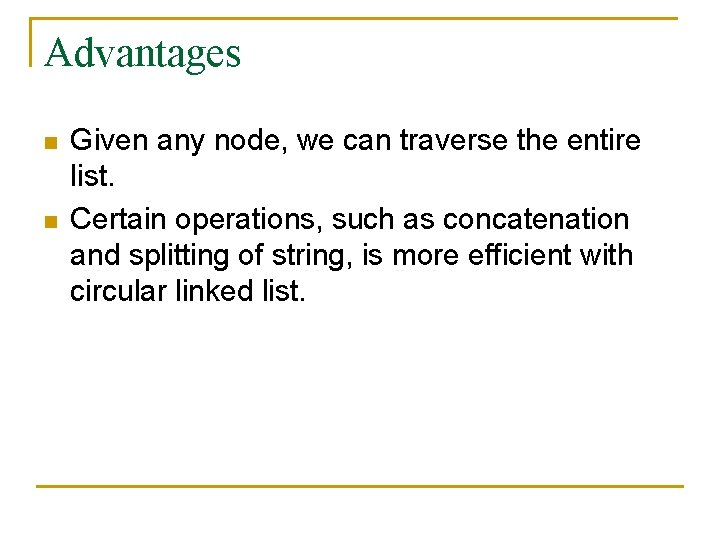Advantages n n Given any node, we can traverse the entire list. Certain operations, such as concatenation and splitting of string, is more efficient with circular linked list.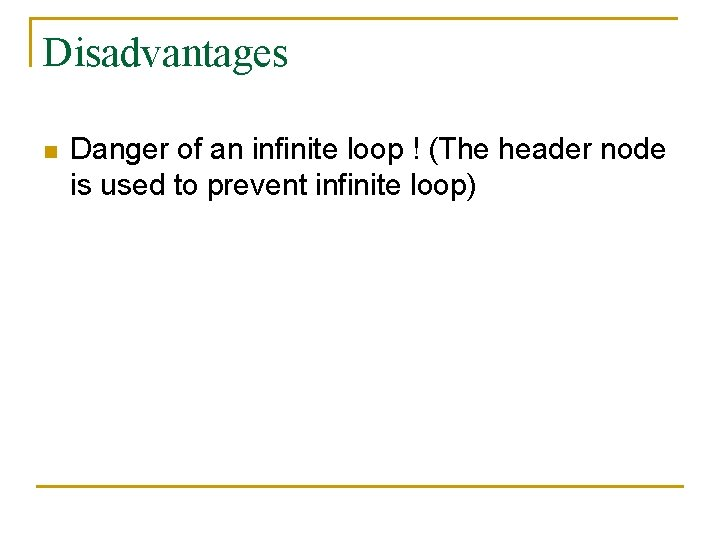Disadvantages n Danger of an infinite loop ! (The header node is used to prevent infinite loop)C Implementation n The structure definition of the circular linked lists and the linear linked list is the same: struct Node. C { int info; struct Node. C* nextptr; }; typedef struct Node. C; Node. C *first = NULL; Node. C *rear = NULL;C Implementation … //Function to insert at beginning in a circular linked list void insert. Beg(int item) { Node. C *ptr = (Node. C *)malloc(sizeof(Node. C)); ptr->info = item; if(first == NULL) { ptr->next = ptr; first = rear = ptr; } else { ptr->next = first; rear->next = ptr; first = ptr; } }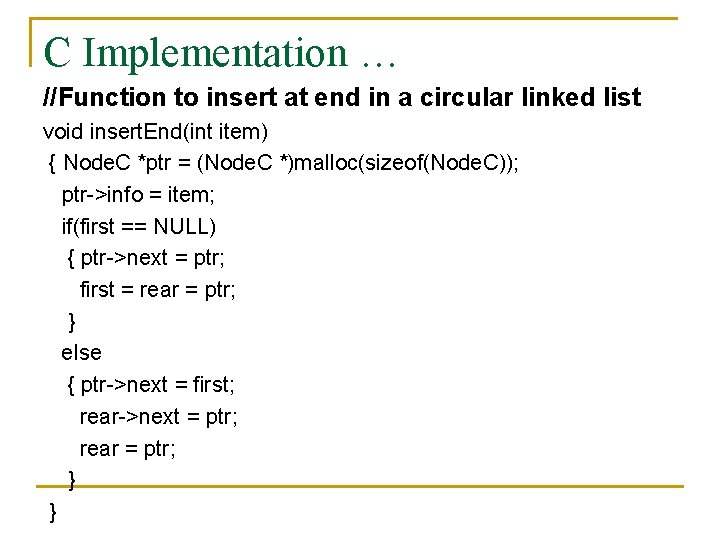C Implementation … //Function to insert at end in a circular linked list void insert. End(int item) { Node. C *ptr = (Node. C *)malloc(sizeof(Node. C)); ptr->info = item; if(first == NULL) { ptr->next = ptr; first = rear = ptr; } else { ptr->next = first; rear->next = ptr; rear = ptr; } }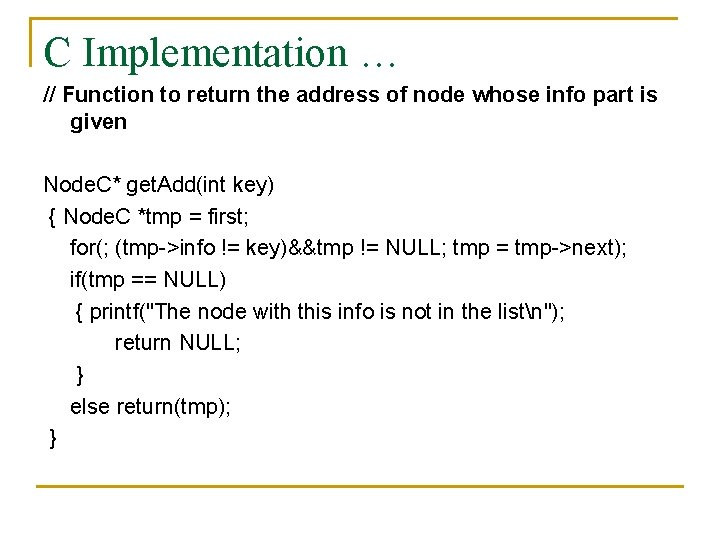C Implementation … // Function to return the address of node whose info part is given Node. C* get. Add(int key) { Node. C *tmp = first; for(; (tmp->info != key)&&tmp != NULL; tmp = tmp->next); if(tmp == NULL) { printf("The node with this info is not in the listn"); return NULL; } else return(tmp); }C Implementation … // Function to insert a node after a given node, whose info is given void insert. Loc(int item, int key) { Node. C *ptr = (Node. C*)malloc(sizeof(Node. C)); Node. C *loc = get. Add(key); ptr->info = item; ptr->next = loc->next; loc->next = ptr; }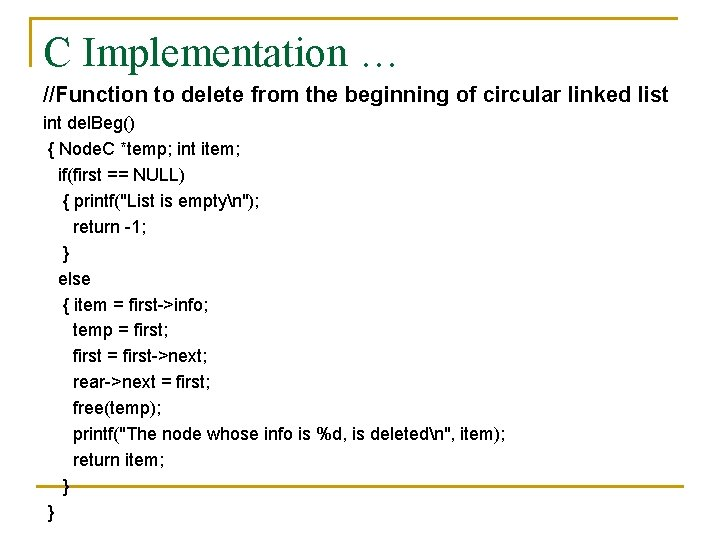C Implementation … //Function to delete from the beginning of circular linked list int del. Beg() { Node. C *temp; int item; if(first == NULL) { printf("List is emptyn"); return -1; } else { item = first->info; temp = first; first = first->next; rear->next = first; free(temp); printf("The node whose info is %d, is deletedn", item); return item; } }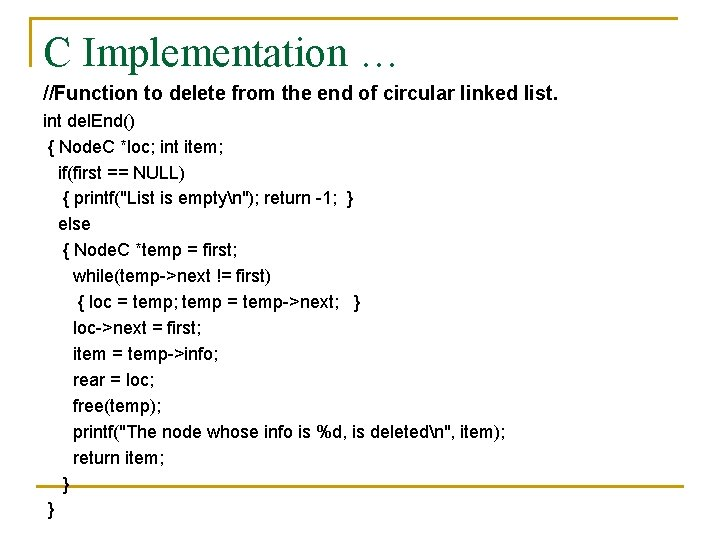C Implementation … //Function to delete from the end of circular linked list. int del. End() { Node. C *loc; int item; if(first == NULL) { printf("List is emptyn"); return -1; } else { Node. C *temp = first; while(temp->next != first) { loc = temp; temp = temp->next; } loc->next = first; item = temp->info; rear = loc; free(temp); printf("The node whose info is %d, is deletedn", item); return item; } }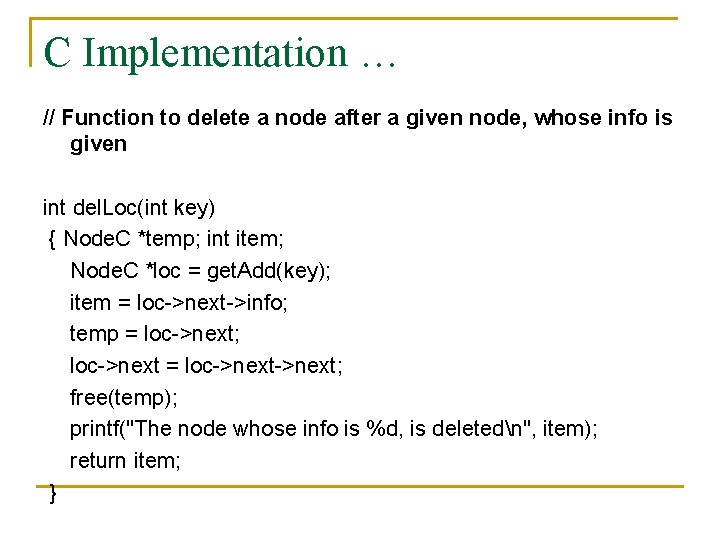C Implementation … // Function to delete a node after a given node, whose info is given int del. Loc(int key) { Node. C *temp; int item; Node. C *loc = get. Add(key); item = loc->next->info; temp = loc->next; loc->next = loc->next; free(temp); printf("The node whose info is %d, is deletedn", item); return item; }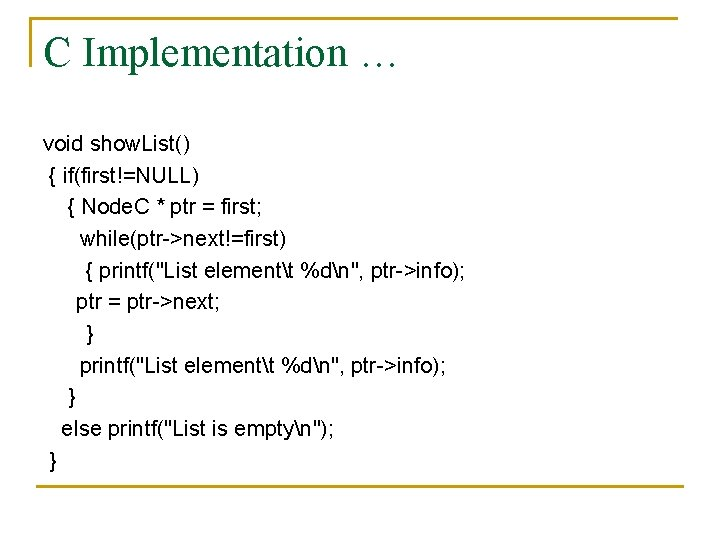C Implementation … void show. List() { if(first!=NULL) { Node. C * ptr = first; while(ptr->next!=first) { printf("List elementt %dn", ptr->info); ptr = ptr->next; } printf("List elementt %dn", ptr->info); } else printf("List is emptyn"); }Deleting a node from the list n n n To delete a node in the end, traverse the list till the last node is reached using temporary pointer p. The pointer is loc made to point the node before the node which is to be deleted. loc->next=p->next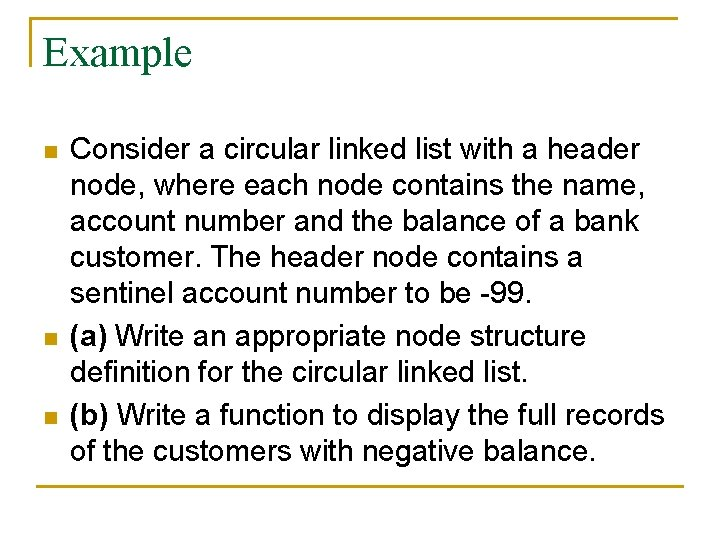Example n n n Consider a circular linked list with a header node, where each node contains the name, account number and the balance of a bank customer. The header node contains a sentinel account number to be -99. (a) Write an appropriate node structure definition for the circular linked list. (b) Write a function to display the full records of the customers with negative balance.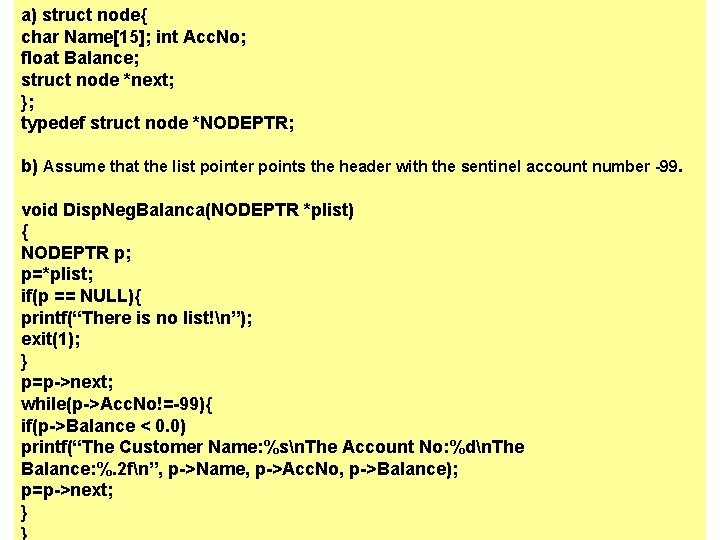a) struct node{ char Name; int Acc. No; float Balance; struct node *next; }; typedef struct node *NODEPTR; b) Assume that the list pointer points the header with the sentinel account number -99. void Disp. Neg. Balanca(NODEPTR *plist) { NODEPTR p; p=*plist; if(p == NULL){ printf(“There is no list!n”); exit(1); } p=p->next; while(p->Acc. No!=-99){ if(p->Balance < 0. 0) printf(“The Customer Name: %sn. The Account No: %dn. The Balance: %. 2 fn”, p->Name, p->Acc. No, p->Balance); p=p->next; } }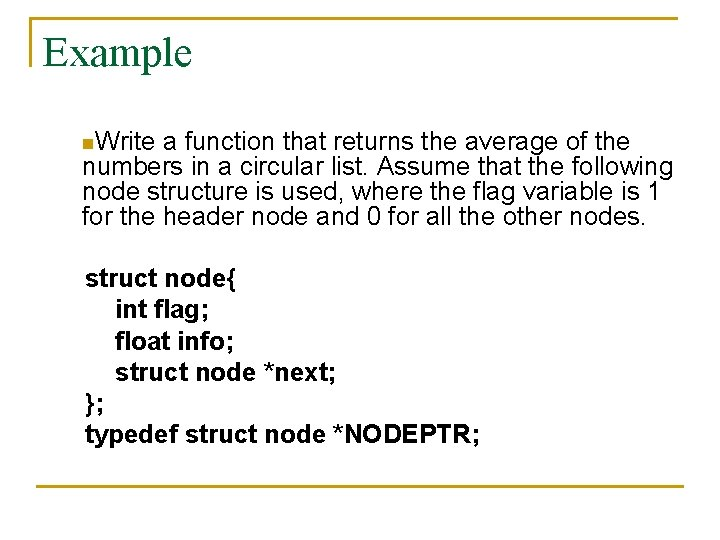Example n. Write a function that returns the average of the numbers in a circular list. Assume that the following node structure is used, where the flag variable is 1 for the header node and 0 for all the other nodes. struct node{ int flag; float info; struct node *next; }; typedef struct node *NODEPTR;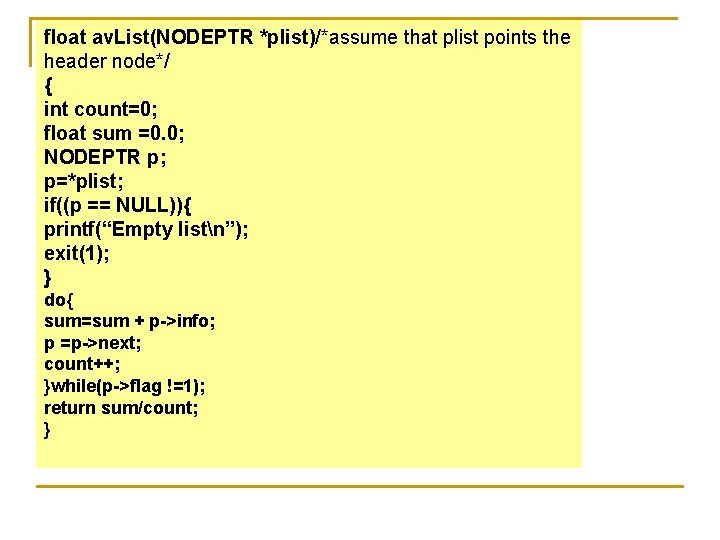float av. List(NODEPTR *plist)/*assume that plist points the header node*/ { int count=0; float sum =0. 0; NODEPTR p; p=*plist; if((p == NULL)){ printf(“Empty listn”); exit(1); } do{ sum=sum + p->info; p =p->next; count++; }while(p->flag !=1); return sum/count; }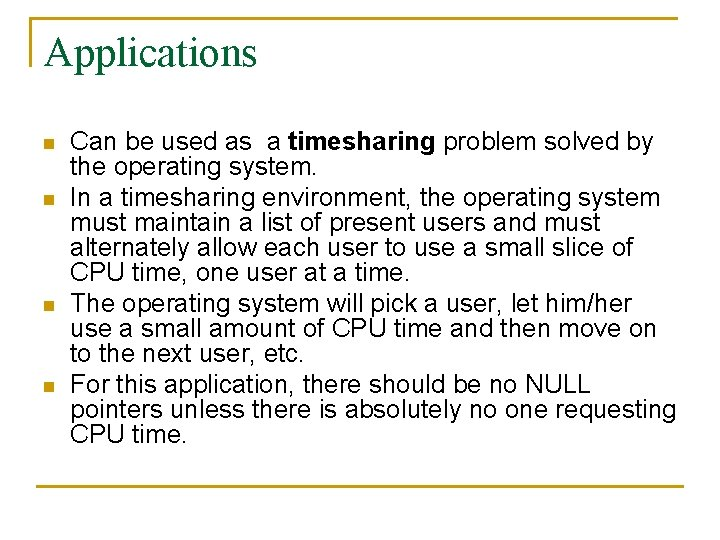Applications n n Can be used as a timesharing problem solved by the operating system. In a timesharing environment, the operating system must maintain a list of present users and must alternately allow each user to use a small slice of CPU time, one user at a time. The operating system will pick a user, let him/her use a small amount of CPU time and then move on to the next user, etc. For this application, there should be no NULL pointers unless there is absolutely no one requesting CPU time.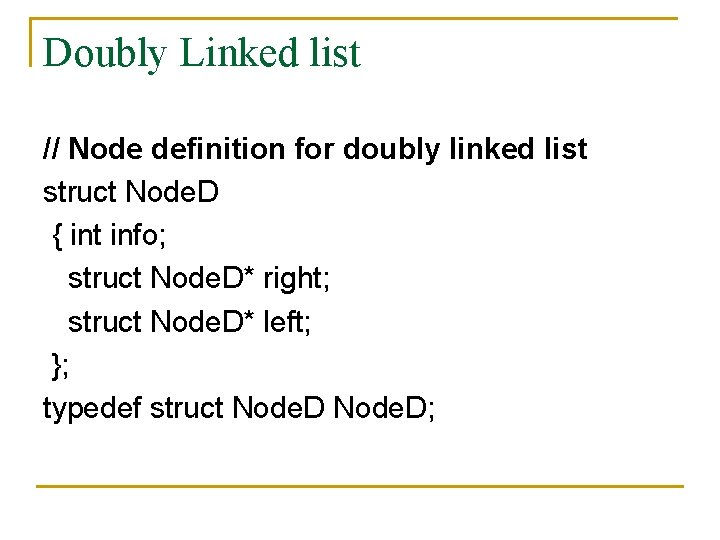Doubly Linked list // Node definition for doubly linked list struct Node. D { int info; struct Node. D* right; struct Node. D* left; }; typedef struct Node. D;Doubly Linked list … Insertion n We visualize operation insert. After(p, X), which returns position q p A B C p A q B C X p A q B X CDoubly Linked list … //Function to insert a Node. D at the beginning of the linked list Node. D* insert. Beg(int item, Node. D* head) { Node. D *ptr = (Node. D*)malloc(sizeof(Node. D)); ptr->info = item; ptr->left = head; ptr->right = head->right; head->right->left = ptr; head->right = ptr; return head; }Doubly Linked list … //Function to insert a Node. D at the end of the linked list Node. D* insert. End(int item, Node. D* head) { Node. D *ptr = (Node. D *)malloc(sizeof(Node. D)); ptr->info = item; ptr->left = head->left; ptr->right = head; head->left->right = ptr; head->left = ptr; return head; }Doubly Linked list … // Function to return the address of Node. D whose info part is given Node. D* get. Add(int key, Node. D *head) { Node. D *tmp = head->right; if(tmp != head) { for(; (tmp->info != key) && (tmp!=head); tmp = tmp->right); return tmp; } else { printf("The Node. D with this info is not in the listn"); return NULL; } }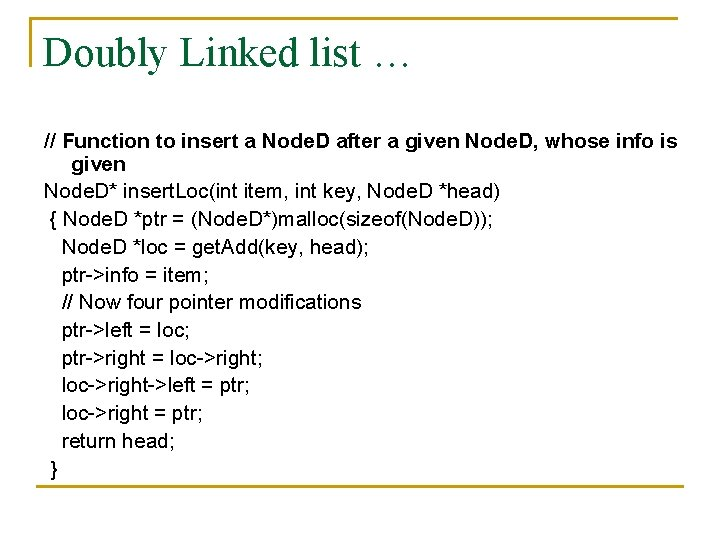Doubly Linked list … // Function to insert a Node. D after a given Node. D, whose info is given Node. D* insert. Loc(int item, int key, Node. D *head) { Node. D *ptr = (Node. D*)malloc(sizeof(Node. D)); Node. D *loc = get. Add(key, head); ptr->info = item; // Now four pointer modifications ptr->left = loc; ptr->right = loc->right; loc->right->left = ptr; loc->right = ptr; return head; }Deletion n We visualize remove(p), where p == last() A B C p D A B C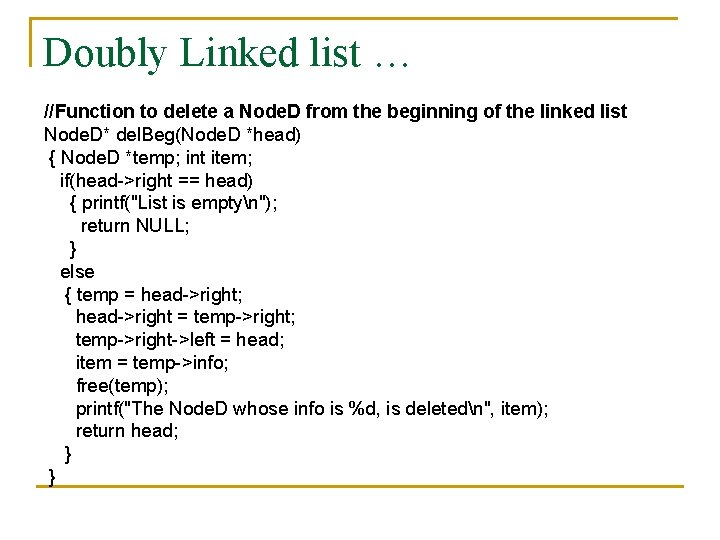Doubly Linked list … //Function to delete a Node. D from the beginning of the linked list Node. D* del. Beg(Node. D *head) { Node. D *temp; int item; if(head->right == head) { printf("List is emptyn"); return NULL; } else { temp = head->right; head->right = temp->right; temp->right->left = head; item = temp->info; free(temp); printf("The Node. D whose info is %d, is deletedn", item); return head; } }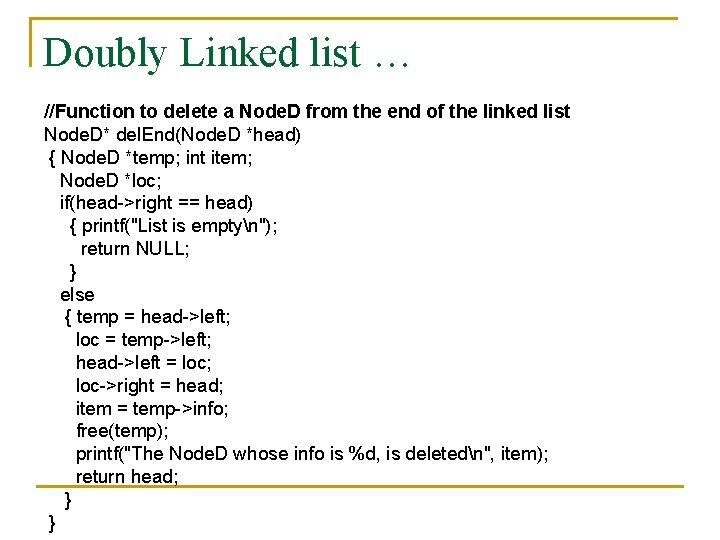Doubly Linked list … //Function to delete a Node. D from the end of the linked list Node. D* del. End(Node. D *head) { Node. D *temp; int item; Node. D *loc; if(head->right == head) { printf("List is emptyn"); return NULL; } else { temp = head->left; loc = temp->left; head->left = loc; loc->right = head; item = temp->info; free(temp); printf("The Node. D whose info is %d, is deletedn", item); return head; } }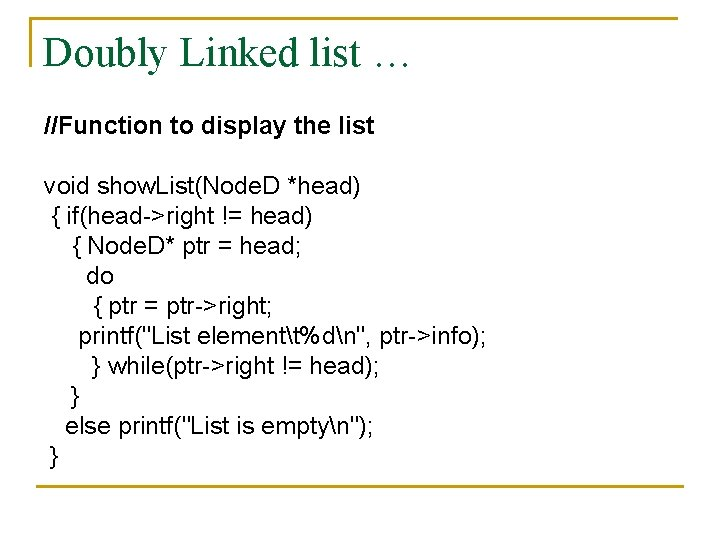Doubly Linked list … //Function to display the list void show. List(Node. D *head) { if(head->right != head) { Node. D* ptr = head; do { ptr = ptr->right; printf("List elementt%dn", ptr->info); } while(ptr->right != head); } else printf("List is emptyn"); }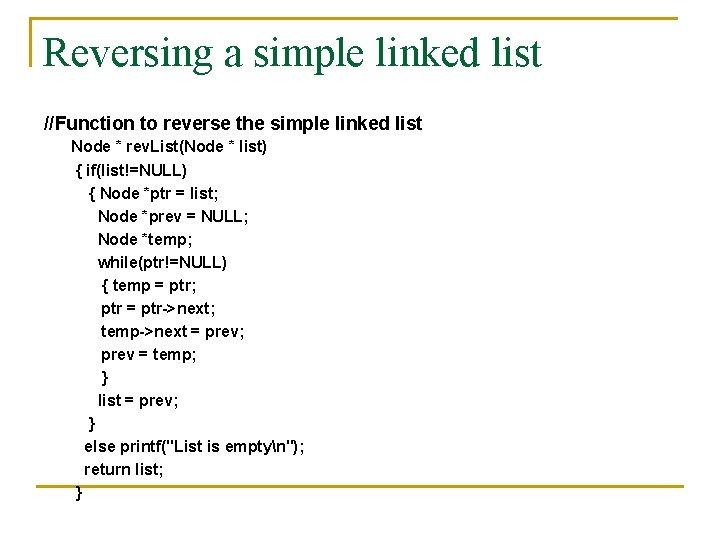Reversing a simple linked list //Function to reverse the simple linked list Node * rev. List(Node * list) { if(list!=NULL) { Node *ptr = list; Node *prev = NULL; Node *temp; while(ptr!=NULL) { temp = ptr; ptr = ptr->next; temp->next = prev; prev = temp; } list = prev; } else printf("List is emptyn"); return list; }